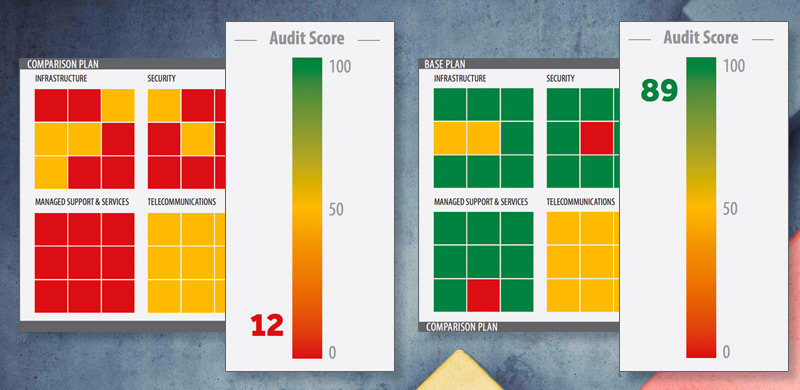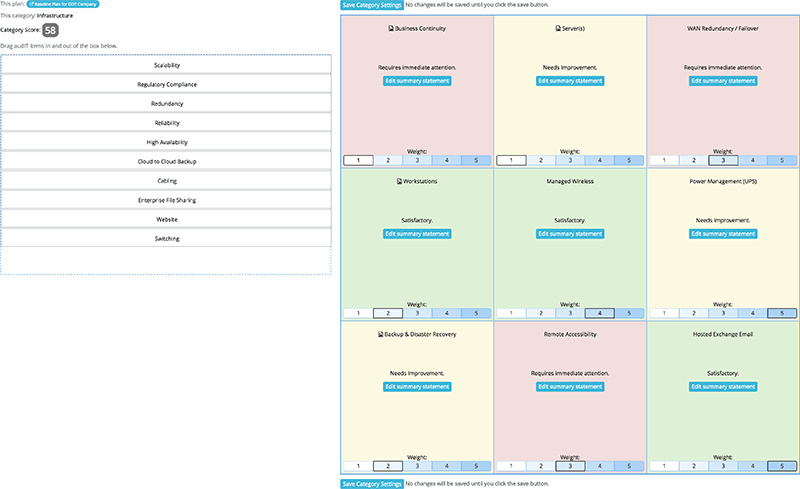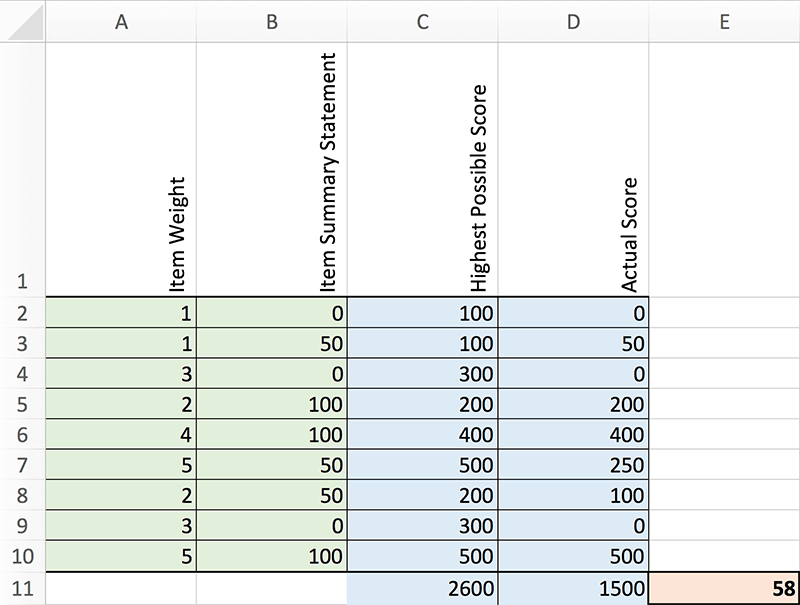# How Is The Audit Score Calculated?

June 25th, 2016When you’re ready to show a prospect the deficiencies in their IT environment, the audit score will be your ally.

It will show, on a scale of 0-100, the health of their environment and give them an immediate visual that will have an emotional impact. Imagine showing your prospect a score of 23 out of 100, for example. Without any explanation from you, they can immediately see that there is a real problem.

Here’s how the audIT score works.

### Understanding The Audit Setup

An audit report is made up of four quadrants. Three of them are mandatory and they include Infrastructure, Security, and Managed Support & Services. The fourth category, Telecommunications, is optional, and can be included or excluded depending on whether it is relevant to your solution stack.

The audit score is calculated based on the three primary quadrants: Infrastructure, Security, and Managed Support & Services. It does not include Telecommunications, which is scored on its own.

Within each quadrant, you’ll select nine audit items and then assign a relative weight to each item on a scale of one to five, depending on each item’s importance to your client or prospect. For example, Backup & Disaster recovery may represent a five out of five in importance. But perhaps Cabling only represents a two out of five.

The weighting plays a key role in the overall audit score, which we’ll get to in a moment, so choose wisely based on your client’s or prospect’s needs.

Once you conduct the audit, enter your results as one of three possible outcomes: satisfactory (green), needs improvement (yellow) or requires immediate attention (red).

The audit score is then calculated based on your item weights and scoring outcomes.

Here’s an example of an audIT that already has weights and outcomes applied. We’ll use this example in the math below.### The Math Behind The Scoring Outcomes

Each color is assigned a numeric value within the system. Red equals 0, yellow equals 50, and green equals 100.

First, the highest possible score is calculated for each individual audit item. This is determined by multiplying 100 (green) by the item weight (1 to 5). This represents your best case scenario for each item.

For example, the highest possible score for an item that you weighted at a 2 would be 200. The highest possible score for an item weighted at a 5 would be 500.

Next, the actual score is calculated for each individual audit item. This is determined by multiplying the item outcome (0, 50 or 100) by its weight.

For example, if your outcome for an item weighted at a 2 was red, the score would be 2 (weight) x 0 (outcome). If your outcome for that same item was yellow, the score would be 2 (weight) x 50 (outcome).

Behind the scenes, there are now two sets of numbers: the highest possible score for each item and the actual score for each item.

Then highest possible scores within each quadrant are totaled, resulting in a total highest possible score for each quadrant.

The actual scores are then totaled in the same way, resulting in a total actual score for each quadrant.

The quadrant score is then calculated by taking the actual score and dividing it by the highest possible score and multiplying by 100. When you’re done, you’ll have an audit score for each quadrant.

Finally, the same formula is applied to reach the overall audit score, where the highest possible score is calculated for each quadrant, the actual score is calculated for each quadrant, the quadrant totals are summed and then the actual score is divided by the highest possible score and multiplied by 100.

Here’s how the math looks in a spreadsheet across a single quadrant with 9 audit items.The important thing to remember with the audit score is that your prospects will be able to immediately visualize the extent of the deficiencies in their IT environment. And your clients will be able to see how taking your recommendations and implementing new solutions improves their score over time.